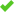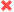翻译您的网站网站翻译变得简单

GTranslate可以翻译任何HTML网站，并使其成为多语言。 它将帮助您增加国际流量，吸引全球观众，开拓新市场。

尝试使用免费的15日试验GTranslate

• 0+ 十亿 翻译页面服务
• 0+ 百万 免费插件下载
• 0+ 兆字节 翻译过去一个月
• 0 活跃客户搜索引擎索引搜索引擎友好机器翻译翻译编辑使用统计语言托管网址翻译15日无风险试用

Free

\$ 0• 语言选择器小部件
•所有语言
•机器翻译
•无限的单词和综合浏览量
•搜索引擎索引
•编辑翻译
•网址翻译
•语言托管

Custom

\$ 5
.99 /月Startup

\$ 14
.99 /月• 翻译交付网络
•所有语言
•神经翻译
•无限的单词和综合浏览量
•搜索引擎索引
•编辑翻译
•网址翻译
•语言托管

Enterprise

\$ 34
.99 /月• 翻译交付网络
•所有语言
•神经翻译
•无限的单词和综合浏览量
•搜索引擎索引
•编辑翻译
•网址翻译
•语言托管• 南非荷兰语 <-> 英语
• 阿尔巴尼亚人 <-> 英语
• 阿姆哈拉语 <-> 英语
• 阿拉伯语 <-> 英语
• 亚美尼亚 <-> 英语
• Azeerbaijani <-> 英语
• 巴斯克 <-> 英语
• 孟加拉 <-> 英语
• 波斯尼亚 <-> 英语
• 保加利亚语 <-> 英语
• 加泰罗尼亚 <-> 英语
• 宿务 <-> 英语
• 简体中文 <-> 英语
• 中国（繁体） <-> 英语
• 科西嘉 <-> 英语
• 克罗地亚 <-> 英语
• 捷克 <-> 英语
• 丹麦语 <-> 英语
• 荷兰人 <-> 英语
• 爱沙尼亚语 <-> 英语
• 芬兰 <-> 英语
• 法文 <-> 英语
• 加利亚西语 <-> 英语
• 格鲁吉亚语 <-> 英语
• 德语 <-> 英语
• 希腊语 <-> 英语
• 古吉拉特语 <-> 英语
• 希伯来语 <-> 英语
• 印地语 <-> 英语
• 匈牙利 <-> 英语
• 北日耳曼语 <-> 英语
• 印度尼西亚人 <-> 英语
• 意大利语 <-> 英语
• 日本性玩偶 <-> 英语
• 爪哇 <-> 英语
• 卡纳达语 <-> 英语
• 哈萨克人 <-> 英语
• 高棉 <-> 英语
• 韩语 <-> 英语
• 库尔德人 <-> 英语
• 拉脱维亚 <-> 英语
• 立陶宛 <-> 英语
• 马其顿 <-> 英语
• 马来语 <-> 英语
• 马拉雅拉姆语 <-> 英语
• 马拉 <-> 英语
• 蒙古人 <-> 英语
• 挪威语 <-> 英语
• Nyanja（奇切瓦） <-> 英语
• 普什图语 <-> 英语
• 波斯语 <-> 英语
• 波兰语 <-> 英语
• 葡萄牙语 <-> 英语
• 旁遮普 <-> 英语
• 罗马尼亚 <-> 英语
• 俄语 <-> 英语
• 塞尔维亚 <-> 英语
• 塞索托语 <-> 英语
• 绍纳语 <-> 英语
• 信德 <-> 英语
• 僧伽罗人 <-> 英语
• 斯洛伐克 <-> 英语
• 斯洛文尼亚 <-> 英语
• 西班牙语 <-> 英语
• 斯瓦希里 <-> 英语
• 瑞典语 <-> 英语
• 菲律宾语（菲律宾语） <-> 英语
• 泰米尔人 <-> 英语
• 泰卢固语 <-> 英语
• 泰国人 <-> 英语
• 土耳其 <-> 英语
• 乌克兰 <-> 英语
• 乌尔都语 <-> 英语
• 乌兹别克 <-> 英语
• 越南人 <-> 英语
• 科萨 <-> 英语
• 祖鲁 <-> 英语• Custom
• Enterprise
• 清除地理位置定位
• 最适合搜索引擎优化
• 容易分离的场地
• 没有服务器要求
• 昂贵
• 严格的ccTLD要求（有时）

• Custom
• Startup
• Enterprise
• 更好的地理位置定位
• 对SEO很好
• 容易分离的场地
• 没有服务器要求
• 用户可能无法从URL中识别地理位置定位

• Custom
• Startup
• Enterprise
• 良好的地理位置定位
• 对SEO很好
• 用户可能无法从URL中识别地理位置定位
• 没有分离的网站
• 您的服务器需要PHP
URL参数 example.com?lang=de 不可用
• 不建议
• 基于URL的分段困难
• 用户可能无法从URL中识别地理位置定位
• 快速设置
• 没有服务器要求
• 没有在搜索引擎索引
• 没有SEO优势GTranslate TDN技术是一种基于云的网站翻译方法。 无需更改您的环境并安装软件来本地化您的网站。 您的多语言网站可以在5分钟内运行。GTranslate可以翻译网站URL，这是多语言SEO的重要组成部分。 下面是一个例子15日免费试用如何工作？